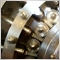# Check My Code10466

wish that my code was working in tester, it seems to be stuck in a loop. im new to using a while loop in the start/on tick and was hoping for anyone familiar with this ..the while loop is for waiting up to 15 seconds for spread ( with each time frame ) to be normal. so basically there is no way in tester to run something such as this? my 1st guess was in making a tick log and i'd rather use something smarter

```extern string Tip1_MoneyManagement = "100.00 (Equity) * 0.0001 (Risk) = 0.01 (Lot)";
extern string Tip2_MoneyManagement = "10,000.00 (Equity) * 0.0001 (Risk) = 1.0 (Lot)";
static datetime W1Bar1Time;
static datetime D1Bar1Time;
static datetime H4Bar1Time;
int iDateTime;
string sSymbol;
int iTicket;
int iType;
extern double dRisk          = 0.0001;
double dLotSize;
double dPrice;
extern    int iSlippage      = 2;
double dSL;
double dTP;
string sComment;
color cArrow;
int iMultiplier;
double dSpread;
extern double dMaxSpread     = 3.0;
extern string Tip3_CurrenciePairs = "EURUSD, GBPUSD, USDJPY, USDCAD,";
extern string Tip4_CurrenciePairs = "EURGBP, EURCHF, EURJPY, AUDUSD,";
extern string Tip5_CurrenciePairs = "CADJPY, AUDNZD, AUDJPY, NZDUSD.";
//+-------------------------------------------------------------------+
int OnInit()
{
sSymbol=Symbol();
if(Digits==3){iMultiplier=100;}
if(Digits==5){iMultiplier=10000;}
//skip first bar
if(H4Bar1Time==""){H4Bar1Time=iTime(sSymbol,240,1);}
if(D1Bar1Time==""){D1Bar1Time=iTime(sSymbol,1440,1);}
if(W1Bar1Time==""){W1Bar1Time=iTime(sSymbol,10080,1);}
return(INIT_SUCCEEDED);
}
//+-------------------------------------------------------------------+
void OnTick()
{

if(H4Bar1Time!=iTime(sSymbol,240,1))
{
H4Bar1Time=iTime(sSymbol,240,1);
iDateTime=TimeCurrent();
while(TimeCurrent()-iDateTime <= 15)
{
dSpread=NormalizeDouble((Ask-Bid)*iMultiplier,1);
if(SignalOn(240) && dSpread<=dMaxSpread)
{
if(PlaceOrder(240)){break;}
}
}
}

if(D1Bar1Time!=iTime(sSymbol,1440,1))
{
D1Bar1Time=iTime(sSymbol,1440,1);
iDateTime=TimeCurrent();
while(TimeCurrent()-iDateTime <= 15)
{
dSpread=NormalizeDouble((Ask-Bid)*iMultiplier,1);
if(SignalOn(1440) && dSpread<=dMaxSpread)
{
if(PlaceOrder(1440)){break;}
}
}
}

if(W1Bar1Time!=iTime(sSymbol,10080,1))
{
W1Bar1Time=iTime(sSymbol,10080,1);
iDateTime=TimeCurrent();
while(TimeCurrent()-iDateTime <= 15)
{
dSpread=NormalizeDouble((Ask-Bid)*iMultiplier,1);
if(SignalOn(10080) && dSpread<=dMaxSpread)
{
if(PlaceOrder(10080)){break;}
}
}
}

}
```24704

Subgenius: wish that my code was working in tester, it seems to be stuck in a loop. i
You can't loop in the tester; you must return to get the next tick.10466

WHRoeder:
You can't loop in the tester; you must return to get the next tick.

Okay, that must be it but its true other than i price calls

my feelings about mt5 is a good one, maybe i can go mtf in tester with loops and all that, well idk yet

check me out: https://www.mql5.com/en/users/subgenius